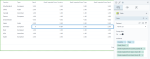# DCPY.IMPUTER(strategy, column(s))

The DCPY.IMPUTER function inserts missing values in a column based on the selected strategy parameter. When two columns are selected, the first one is the column to impute, and the second one will be used as the category column, where for each category the selected strategy is calculated.

###### Parameters
• strategy – Strategy to use for imputation. The strategy is ignored when the column to impute is of a character type, which is always imputed with the most_frequent strategy.
• mean – Replace missing values using the average of the column.
• median – Replace missing values using the median of the column.
• most_frequent – Replace missing values using the most frequent value occurring in the column.
• column(s) – Dataset column or custom calculation.

Example: DCPY.IMPUTER(‘mean’, sum([Rank]), [Country]).

###### Input data

You can include one of the following:

• One numeric or character column that contains missing values to impute.
• A numeric and a character column, where the first numeric column to impute is based on the category summary of the second column.
• Two character columns, where the first column to impute is based on its most frequent value by category in the second column.
###### Result
• The original input column that includes imputed missing values.
###### Key usage points
• Use it when you need to replace missing values to retain the whole data set in case of limited data.
###### Example

In the following example, we have one missing value in the Rank column for Germany. To impute the missing rank value, we added three imputer calculations with different parameters:

• DCPY.IMPUTER('mean', sum([Rank]), [Country]) — The function returned “N.aN” because the Country column does not have another value for Germany and there is no sufficient data

for the imputer.

• DCPY.IMPUTER('mean', sum([Rank]), [Type]) — The function inserted the value of “2” based on the mean for the values of the Type column (total of 8 divided by 4 rows with “European”).

• DCPY.IMPUTER('mean', sum([Rank])) — The function inserted the value of “2” based on the values in the Rank column (total of 20 divided by 10 rows).For the whole list of algorithms, see Data science built-in algorithms.